# jbrry / pytorch-struct

Fast, general, and tested differentiable structured prediction in PyTorch

http://harvardnlp.github.io/pytorch-struct

Geek Repo

Github PK Tool

# Pytorch-Struct

A library of tested, GPU implementations of core structured prediction algorithms for deep learning applications.

• HMM / LinearChain-CRF
• HSMM / SemiMarkov-CRF
• Dependency Tree-CRF
• PCFG Binary Tree-CRF
• ...

Designed to be used as efficient batched layers in other PyTorch code.

## Getting Started

```!pip install -qU git+https://github.com/harvardnlp/pytorch-struct
# Optional CUDA kernels for FastLogSemiring
!pip install -qU git+https://github.com/harvardnlp/genbmm
# For plotting.
!pip install -q matplotlib```
```import torch
from torch_struct import DependencyCRF, LinearChainCRF
import matplotlib.pyplot as plt
def show(x): plt.imshow(x.detach())```
```# Make some data.
vals = torch.zeros(2, 10, 10) + 1e-5
vals[:, :5, :5] = torch.rand(5)
vals[:, 5:, 5:] = torch.rand(5)
dist = DependencyCRF(vals.log())
show(dist.log_potentials)```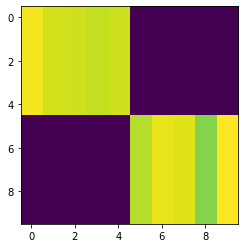```# Compute marginals
show(dist.marginals)```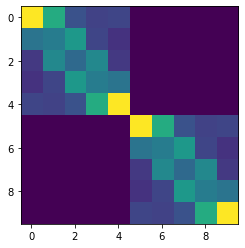```# Compute argmax
show(dist.argmax.detach())```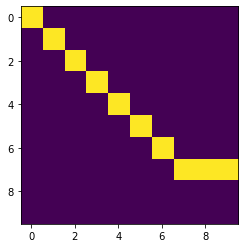```# Compute scoring and enumeration (forward / inside)
log_partition = dist.partition
max_score = dist.log_prob(dist.argmax)```
```# Compute samples
show(dist.sample((1,)).detach()[0, 0])```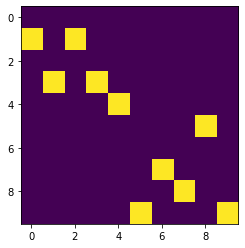```# Padding/Masking built into library.
dist = DependencyCRF(vals, lengths=torch.tensor([10, 7]))
show(dist.marginals)
plt.show()
show(dist.marginals)```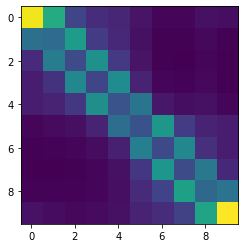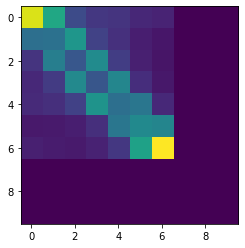```# Many other structured prediction approaches
chain = torch.zeros(2, 10, 10, 10) + 1e-5
chain[:, :, :, :] = vals.unsqueeze(-1).exp()
chain[:, :, :, :] += torch.eye(10, 10).view(1, 1, 10, 10)
chain[:, 0, :, 0] = 1
chain[:, -1,9, :] = 1
chain = chain.log()

dist = LinearChainCRF(chain)
show(dist.marginals.detach().sum(-1))```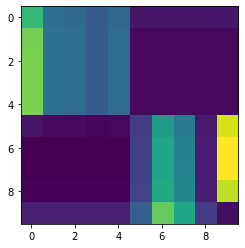## Library

Current distributions implemented:

• LinearChainCRF
• SemiMarkovCRF
• DependencyCRF
• NonProjectiveDependencyCRF
• TreeCRF
• NeuralPCFG / NeuralHMM

Each distribution includes:

• Argmax, sampling, entropy, partition, masking, log_probs, k-max

Extensions:

• Integration with `torchtext`, `pytorch-transformers`, `dgl`
• Adapters for generative structured models (CFG / HMM / HSMM)
• Common tree structured parameterizations TreeLSTM / SpanLSTM

## Low-level API:

Everything implemented through semiring dynamic programming.

• Log Marginals
• Max and MAP computation
• Sampling through specialized backprop
• Entropy and first-order semirings.

## Examples